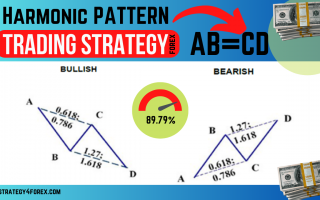# AB=CD Harmonic Pattern [Forex & Crypto Trading Strategy]

The AB=CD Harmonic Pattern [Forex & Crypto Trading Strategy] consists of two equivalent price movements, expressed in terms of Fibonacci ratios.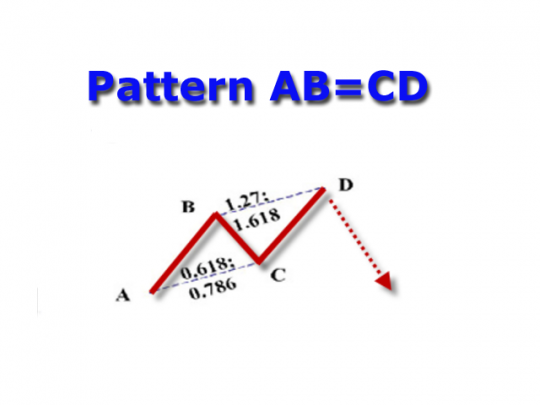This is the basic model, it is often included (in different versions) in some of the other harmonious patterns that we have considered with you on this site:

Very often, especially to adherents of the Elliott wave theory, it may seem that this model is nothing more than a correction to the trend movement, which we also considered earlier — an ABC-type correction.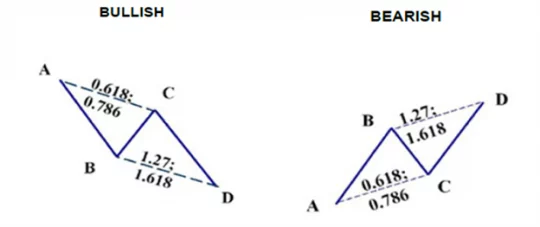This is partly true, but there are many examples when the AB=CD pattern was a reversal pattern, and not a correction, so it cannot be unambiguously considered according to Elliott.

## There are 2 main variants of this pattern, which are presented in Fig. 1 and fig. 2:

On fig. 1 schematically shows the AB=CD pattern with point C retracement equal to 61.8% Fibo. The projection of wave BC in this case is 161.8% Fibonacci.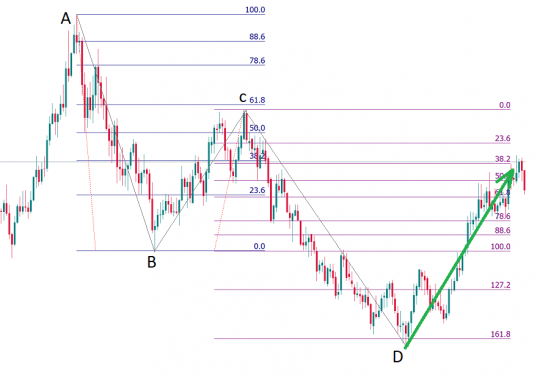A variant of the model with «root» Fibonacci values in fig. 2. The retracement of point C here is 78.6% Fibonacci, and the projection of wave BC is 127.2% Fibo.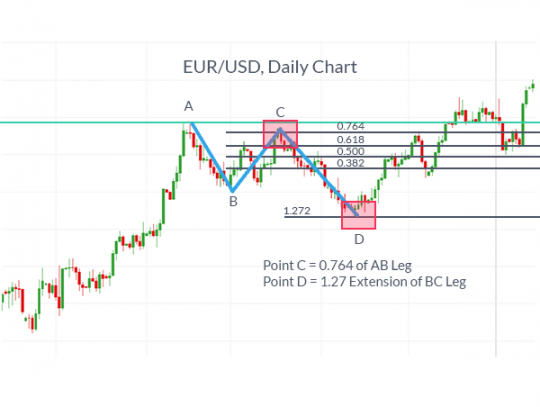But it is also worth knowing that there are models on the market with proportions that differ from those described above. It is not uncommon to see a pattern with a 78.6% Fibonacci retracement and a 161.8% Fibo Extension projection: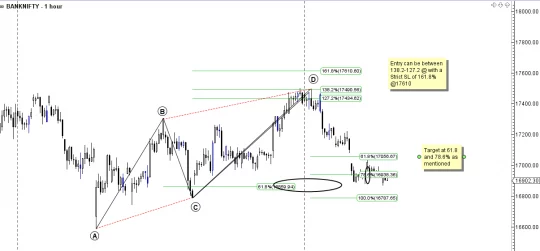### Video with examples: